# Alphabet (computer science) facts for kids

Kids Encyclopedia Facts

In computer science, an alphabet is a finite non-empty set. The elements of an alphabet are called the letters or symbols of the alphabet.

An example of an alphabet is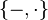$\{-,\cdot\}$ which may be used for Morse code or {begin, if, else, for, while} which may be the keywords of a Programming language.

The set of natural numbers is not an alphabet because as it is not finite.

The alphabet which is used the most in computer science is {0,1}. It is called the binary alphabet because it contains two symbols. An alphabet can be used to make a string (or word). This is a finite Sequence of letters from the alphabet. For example, a string of length 5 over {0,1} is 01101.

The empty string is the string containing no letters (it is often written as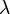$\lambda$). The empty string is a string over any alphabet.

If we have an alphabet called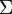$\Sigma$. Then we write the set of all strings that can be made from$\Sigma$ as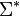$\Sigma^*$. This is called the Kleene star (or Kleene closure) of$\Sigma$. It is named after the mathematician Stephen Cole Kleene.

The Kleene star of the binary alphabet is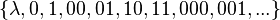$\{\lambda, 0,1,00,01,10,11,000,001,...\}$. The three dots after 001, show that we cannot write the Kleene star of an alphabet in full because it is an infinite set.

Alphabets are important because they are used in studying formal languages, finite automata and very difficult questions in computer science about what can be computed and what can not.

## Related pages

• Arto Salomaa, Formal Languages, (1973): Chapter 1
• Michael A. Harrison, Introduction to Formal Language Theory, (1978): Chapter 1.2
• John E. Hopcroft and Jeffrey D. Ullman, Introduction to Automata Theory, Languages, and Computation, Addison-Wesley Publishing, Reading Massachusetts, (1979). ISBN: 0201-02988-X.
• György E. Révész, Introduction to Formal Languages, (1991): Chapter 1.1
• Grzegorz Rozenberg and Arto Salomaa, Handbook of Formal Languages: Volume 1. Word, Language, Grammar, (1997): Chapter 2.1
• Dan A. Simovici and Richard L. Tenney, Theory of Formal Languages with Applications, (1999): Chapter 2.1
• Keijo Ruohonen, Formal Languages, (2009): Chapter 1.1
• John Martin: Introduction to Languages and the Theory of Computation (2010): Chapter 1.5Alphabet (computer science) Facts for Kids. Kiddle Encyclopedia.